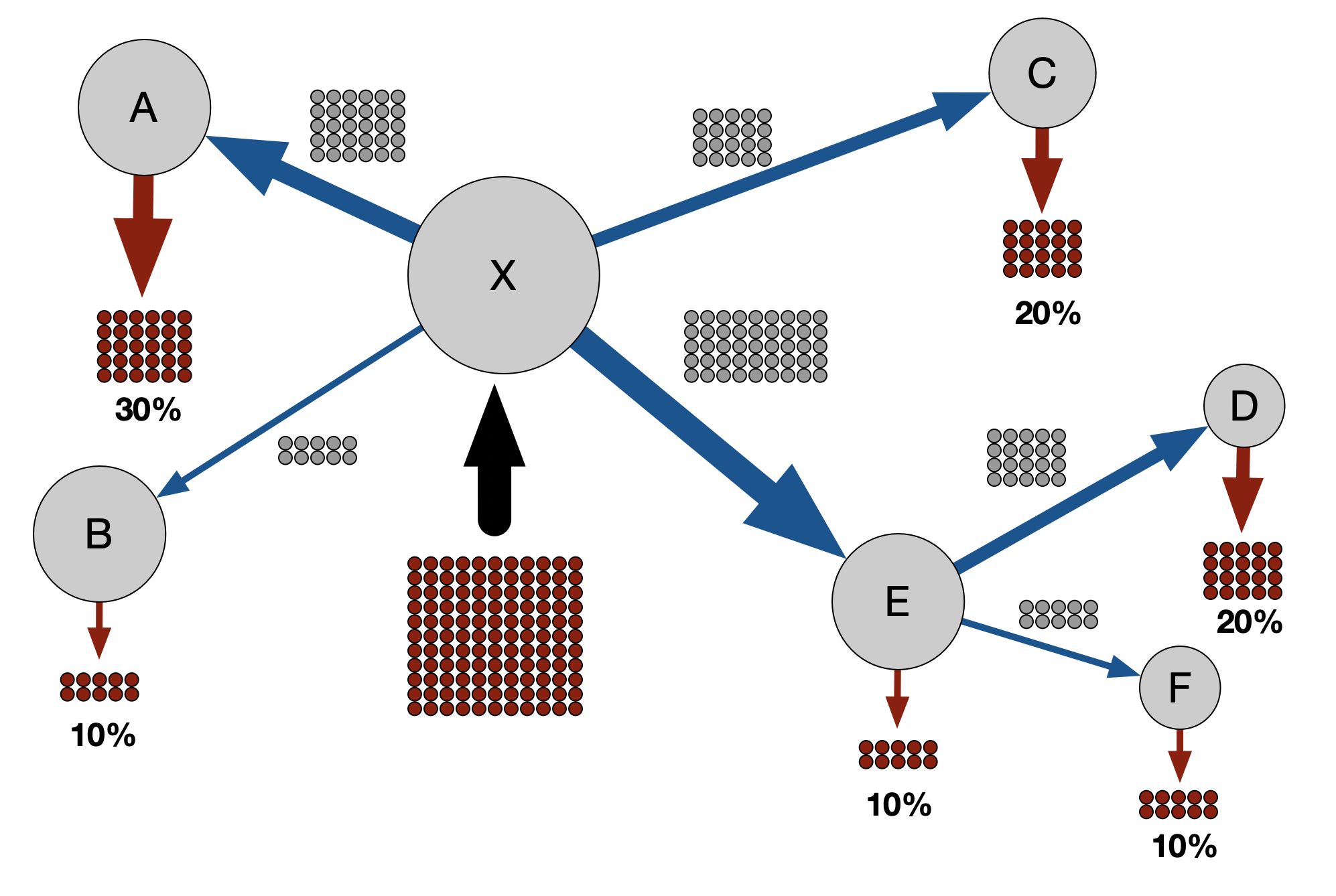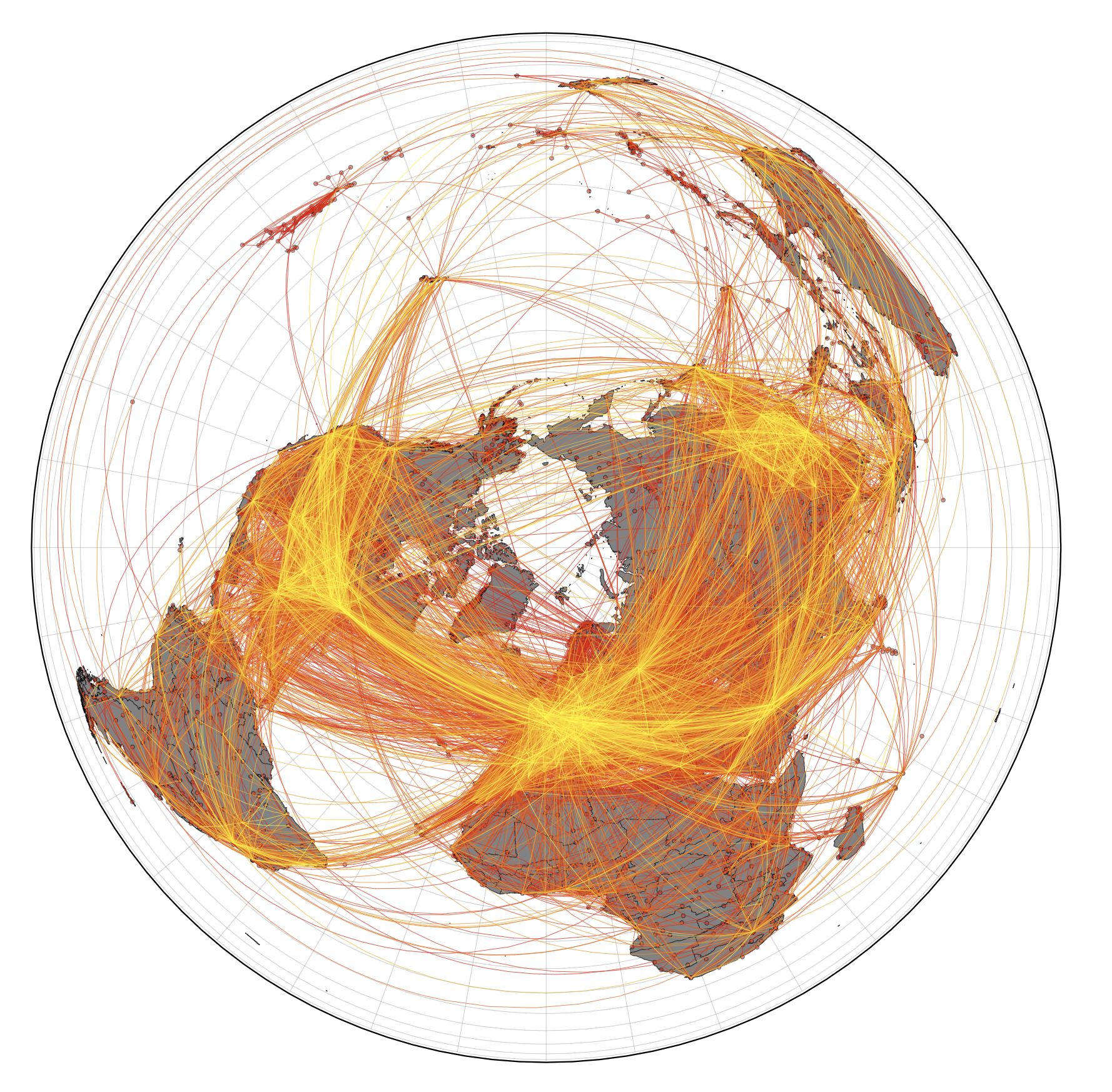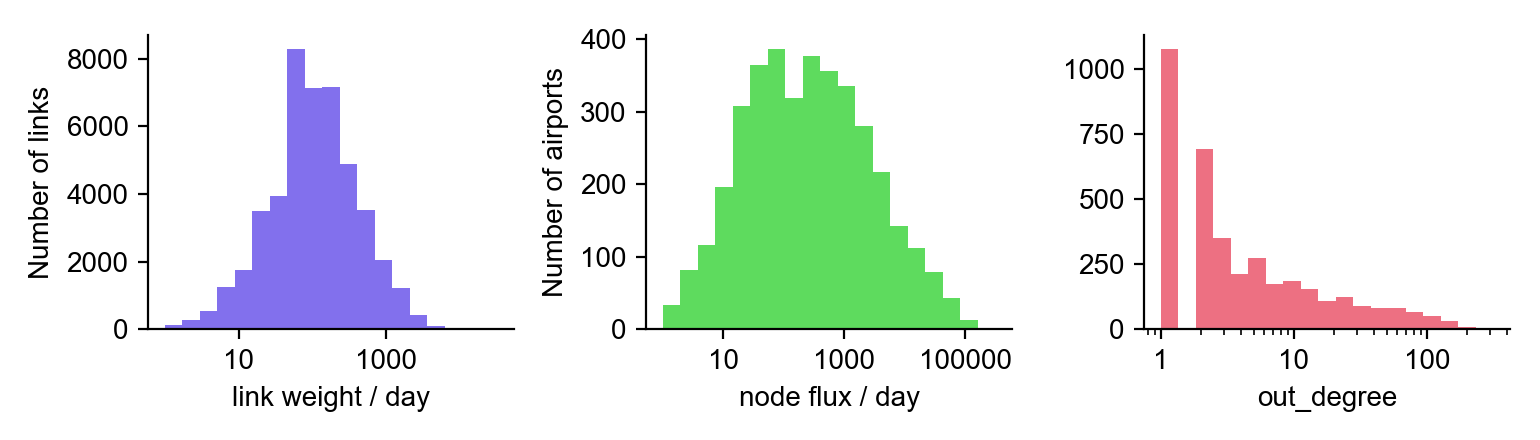# Import Risk: Data and Methods

## Relative Import Risk Explained

If an infected individual boards a plane at airport A in an affected region, the relative import risk P(B|A) at airport B quantifies the probability that airport B is the final destination for that individual (irrespective of non-direct travel routes).

Say, 1000 infected individuals board planes at Johannesburg O.R. Tambo International. An import risk of 0.2% in Germany means that, of those 1000 individuals, only 2 are expected to have Germany as their final destination.Say, 100 infected passengers board planes at airport X en route to destination airports elsewhere in the network, potentially going through transit airports in the process, e.g. passengers traveling to airports D and F in the figure above. The relative import risk at a given destination is simply the fraction of the 100 individuals that entered at airport X and exited at that destination airport. For example, the transit airport E has an import risk of 10% because, even though 40 of the 100 individuals that entered at X traveled to E, only 10 individuals had E as their final destination and exited the network here. The other 30 passengers traveled through E en route to a different destination airport. Transit passengers are depicted in grey.

## Data

Whenever an infectious disease poses the risk of spreading into a global pandemic, air transportation plays a key role. Modern air traffic connects every corner of our globe. In doing so, it also enables regionally confined outbreaks of infectious diseases to “jump” across country borders at an unprecedented rate.

The core of the computational model (explained above, and in detail below) is the worldwide air transporation network (WAN) from August 2021. This network has 3802 nodes (airports) that are connected by 46773 directed links (flight routes). Each link is weighted by the traffic flux between nodes, i.e. the average number of passengers that travel each route per day.

The WAN has been analyzed in many publications and used in a variety of computational models for the spread of infectious diseases.Worldwide air transportation network. This network of global mobility is the foundation of the computational model that predicts import risks, most probable spreading routes, and the importance of specific airports in the global dissemination of COVID-19.

### Properties of the worldwide air transportation network

The WAN exhibits strong intrinsic structural heterogeneity – some airports are tiny, while others are huge international hubs. These distinctions can be observed by examining the wide-range of magnitudes of various properties of the WAN – a clear sign of network heterogeneity, summarized below.Statistics of the worldwide air transportation network. In the first panel of the figure above, the traffic flux betwwen nodes or average daily number of passengers traveling on flight routes between airports (link weight) can vary from less than 1 to over 1000. The second figure shows the number of passengers that traverse an airport on a daily basis (node flux), which does not exceed 100 in some airports, while others accomodate over 10000 people each day. Similarly, in the last panel, the number of flight connections emanating from an airport (out degree) is approx. 13 on average, though some airports boast over 100 direct connections.

The WAN is dominated by big airports. As a result of the network heterogeneity of the WAN, the model is predominantly driven by only a small number of nodes, i.e. the big regional and international hub airports, while the majority of the nodes are structurally trivial. For example, if the smallest half of airports the network (approx. 2000) were removed, only about 7% of the total traffic would be lost.

## Details on the Import Risk Model

In the event of an outbreak of an emergent infectious disease, the most urgent question that arises is how to prevent the possibility of a global pandemic. To address this requires an understanding of the complex and dynamic spreading patterns of the new pathogen, which are largely dominated by air transportation and the import risk it induces. Typically in these situations, there is a small number of affected locations with confirmed cases in addition to an expected, but unknown, number of unconfirmed cases. Initially, we need to understand a situation in which the case number is small (compared to the entire population) and the dynamics are dominated by stochastic events. We are then interested in estimating the import risk without focusing on secondary infections. Due to these initial stochastic assumptions, a model at this stage can only be probabilistic in nature.

During emergent infectious disease situations, such as the Ebola and MERS crises, one of the key questions is:

Given the spatial and regional distribution of confirmed cases, what is the likelihood of importing a case from an affected location to an airport or country distant from the outbreak location?

The core structure of the model is the worldwide air transportation network. The nodes (airports) are labeled $$n=1,…,N.$$ This model estimates the quantity

$$p_{\infty}(m|n_{0}),$$

which is the probability of importing a case at node $m$ from a source airport $n_{0}$.

The air transportation network can be characterized by a flux matrix $\mathbf{F}$ where elements $F_{nm}$ quantify the passenger traffic from node $m$ to $n$. This network has approx. 4000 nodes, 50000 links, and a resulting link density of about 0.3%.

The model outlined below estimates relative import risk based on real traffic. Given a flux matrix $F_{nm}$ between nodes, the model computes the probability that an individual that enters the system at a point of origin $n_{0}$ exits at a target node $n$, potentially traversing intermediate transit nodes in the process. We can write this as

$$F_{nm}\xrightarrow{\text{model}}p_{\infty}(n|n_{0}).$$

### Stochastic network dynamic model

We have a network of $N$ nodes, labeled $n=1,…,N$ that are connected by weighted links $F_{nm}$, where $F_{nm}\geq0$. For now, $F_{nm}$ quantifies the coupling between nodes, which can be interpreted as passenger flux, i.e. individuals that travel from node $m$ to node $n$. Therefore, $F_{nm}$ determines the import risk of an infectious disease from $m$ to $n$, including potential intermediate nodes traversed. This link also facilitates connections to additional nodes, giving rise to potential paths in the network where a substantial fraction of the links are zero. We consider a process in which a passenger enters the system at an node $n_{0}$ (point of entry) and travels along links of the network until they stop at some other node $n$ and exit the network.

First, we define a path $$\Gamma = { n_i } _{i= 0,…,L}$$ as a sequence of $L$ steps along a set of $L+1$ nodes. For each possible path, the process is governed by two probabilities:

#### Transition probability

Given that a person at node $n$ travels to another node, the probability that the next position is $m$ is $P_{nm}$, where $\sum_{n}P_{nm}=1$.

#### Exit probability

The probability that a passenger will exit the system upon arrival at node $n$ is $q_{n}=q(n|n_{0})$, where $0\leq q_{n}\leq1$. The probability that a walker will continue its journey is $1-q_{n}$.

Here, we will make the assumption that this exit probability can also depend on the starting point $n_{0}$ of the walker: $q_{m}=q(m|n_{0})$. However, to keep things uncomplicated, we will implicitly remember this dependency, but not state it explicitly.

We will derive both $P_{nm}$ and $q_{n}$ from the basic flux matrix $F_{nm}$ below, but for now, we will assume they are given such that the probability that a path $\Gamma$ is taken is

$$p(\Gamma) = q_{n_{L}}P_{n_{L}n_{L-1}}(1-q_{n_{L-1}})P_{n_{L-1}n_{L-2}} \times…\times(1-q_{n_{k}})P_{n_{k}n_{k-1}}\times…\$$ $$\times(1-q_{n_{2}})P_{n_{2}n_{1}}(1-q_{n_{1}})P_{n_{1}n_{0}}(1-q_{n_{0}})$$ $$=q_{n_{L}}\prod_{k = 1} ^L S_{n_{k}n_{k-1}},$$

where

$$S_{nm}=P_{nm}(1-q_{m}).$$

We also assume that $q_{n_{0}}=0$ at the initial starting point, but include it in the above formula. Because $q_{n}$ is a function of the entry node, $S$ also has this dependency: $$S_{nm}=S(n|m;n_{0}).$$

To put it simply, this just means that the location of the point of entry can determine which of the possible paths is taken. The path probability satisfies $$1=\sum_{\Gamma\in\Omega}p(\Gamma)$$ because the probability that the passenger takes any of the possible paths (all contained in the set $\Omega$) is unity, meaning it is necessarily bound to occur. Now, we consider the probability that a path $\Gamma$ starts at $n_{0}$ and ends at node $n_{L}$ after $t$ steps, which can be denoted by

$$p(n_{L},t|n_{0})=\sum_{\Gamma\in\Omega(n_{L},n_{0},t)}p(\Gamma)$$ where $\Omega(n_{L},n_{0},t)$ is the subset of paths that begin at $n_{0}$ and end at $n_{L}$ after time $t$. As a result, we have

$$p(n_{L},t|n_{0}) = q_{n_{L}}\sum_{n_{L - 1}}\sum_{n_{L - 2}}…\sum_{n_{2}}\sum_{n_{1}}\left(\prod_{k = 1}^{L}S_{n_{k}n_{k-1}}\right),$$

which can be reduced to

$$p(m,t|n_{0})=q_{m}\times( \mathbf{S} ^t ) _{mn_0}.$$

From the above, we get $$\sum_{m,t = 1} p(m,t|n_{0})=1,$$ allowing us to define

$$p_{\infty}(m|n_{0})=\sum_{t}p(m,t|n_{0}).$$

Note that $p(m,t|n_{0})$ is not the probability that a person is at $m$ at time $t$, but rather the probability that a person exits the system at $m$ at time $t$.

### Connecting the traffic data to the model

In order to derive $P_{nm}$ and $q_{m}$ from $F_{nm}$, we start by saying

$$P_{nm}=F_{nm}/\sum_{k}F_{km}.$$

However, the derivation of $q_{m}$ is more subtle.

### What is $q_{m}$?

The quantity $q_{m}=q(m|n_{0})$ is the probability that once a traveler who started at $n_{0}$ arrives at node $m$ and exits. In a transportation system, typically only the raw flux between locations is known, i.e. the matrix $F_{nm}$. In the air transportation system, we presume that peripheral nodes, e.g. small airports in remote places, have a large $q_{m}$ because passengers that arrive at $m$ will most likely exit there. So we expect $q_{m}\approx1$.

On the other hand, large hub airports that are predominantly used as transit airports have a smaller $q_{m}$ and possibly a stronger dependence on the initial starting point $n_{0}$. In the absence of $q_{m}$ data, we can use air transportation logic to estimate $q_{m}$ in the following way:

First, we assume that a node with total traffic $F_{n}=\sum_{m}F_{mn}$ has a population in the catchment area that is proportional to some power of the population size, denoted as $$N_{n}=aF_{n}^{\xi},$$ where $\xi$ is some scaling exponent. In the simplest case, we can assume that $\xi=1$ giving us

$$N_{n}=aF_{n}.$$ Later, we can generalize the model to $\xi\neq1$. Next, we want to calculate the shortest path tree $T(n_{0})$ by identifying the set of most probable paths originating from a source node $n_{0}$ to all other nodes in the network. This requires a measure of distance associated with each link.

#### Effective distance

Recently, we introduced the notion of effective distance: a probabilistic measure that relates the traffic flux in the network to a distance between connected nodes. We can write this as

$$d_{nm}\sim(d_{0}-\log P_{nm}),$$

where $0\leq d_{0}\leq1$. Here, $d_{0}=1$ defines a distance model in which a path $n_{1}\rightarrow n_{2}\rightarrow n_{3}$ with $P_{n_{1}n_{2}}=P_{n_{2}n_{3}}=1$ is twice the distance as a path $n_{1}\rightarrow n_{3}$, whereas $d_{0}=0$ assigns the same distance to each path.

This allows us to compute the shortest path tree $T(n_{0})$ for a point of origin $n_{0}$. Given a tree $T(n_{0})$, a node $n$ in the tree has a set of nodes $\Omega(n|n_{0})$ that contains the children and offspring of $n$, i.e. all the nodes in the network that start at $n_{0}$ and have paths that go through $n$. The total population in this set is $$N_{n,n_{0}}=\sum_{\omega\in\Omega(n|n_{0})}N_{\omega}.$$ Now, we assume that the exit probability at $n$ is proportional to the ratio of the population at $n$ (i.e. $N_{n}$) to the population of all the offspring $N_{n,n_{0}}$ plus $N_{n}$, such that $$q(n|n_{0})=\frac{N_{n}}{N_{n}+N_{n,n_{0}}}=\frac{F_{n}}{F_{n}+\sum_{\omega\in\Omega(n,n_{0})}F_{\omega}}.$$ By including the node $n$ itself, we have $$q(n|n_{0})=\frac{F_{n}}{\Phi(n|n_{0})},$$ where $$\Phi(n|n_{0})=F_{n}+\sum_{\omega\in\Omega(n|n_{0})}F_{\omega}.$$

Here, $q(n|n_{0})$ depends on the starting node $n_{0}$ because $\Phi(n|n_{0})$ does. The above equations describe the connection between the coupling matrix $F_{nm}$ and the model probabilities $P_{nm}$, as well as $q(n|n_{\text{0}}).$ Note that everything that is part of this connection only depends on the coupling matrix $F_{nm}$ up to a proportionality.